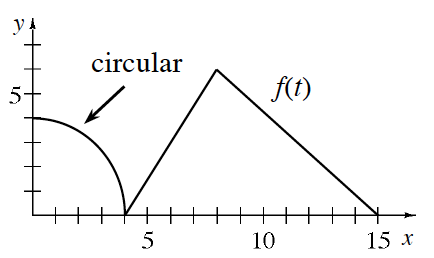### Home > CALC3RD > Chapter Ch7 > Lesson 7.2.2 > Problem7-71

7-71.

$F(x) =\int _ { 0 } ^ { x } f ( t ) d t$ for the function $y = f(t)$ graphed at right.

1. When is $F$ at a maximum on $[0, 15]$?

An integral represents the area under a curve. At which point is the area greatest?

2. Is $F$ increasing, decreasing, or both over the interval $(0, 8)$?

Strictly Increasing. (Justify.)

3. Evaluate $F(4)$, $F(10)$, and $F(15)$.

$F(4)=\int_{0}^{4}f(t)dt=\text{quarter circle}$

$F(10)=\int_{0}^{10}f(t)dt=\text{quarter circle + triangle + trapezoid}$

$F(15)=\int_{0}^{15}f(t)dt=\text{quarter circle + triangle}$

4. List the interval(s) on $[0, 15]$ for which $F^{\prime\prime}(x) > 0$.

Since $F^\prime(x) = f(x)$,
$F^{\prime\prime}(x) = f^\prime(x)$.